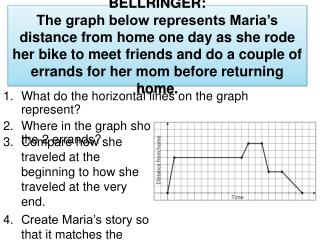DownloadDownload PresentationWhat do the horizontal lines on the graph represent? Where in the graph shows her taking care of the 2 errands?

# What do the horizontal lines on the graph represent? Where in the graph shows her taking care of the 2 errands?

Télécharger la présentation## What do the horizontal lines on the graph represent? Where in the graph shows her taking care of the 2 errands?

- - - - - - - - - - - - - - - - - - - - - - - - - - - E N D - - - - - - - - - - - - - - - - - - - - - - - - - - -
##### Presentation Transcript

1. BELLRINGER:The graph below represents Maria’s distance from home one day as she rode her bike to meet friends and do a couple of errands for her mom before returning home. • What do the horizontal lines on the graph represent? • Where in the graph shows her taking care of the 2 errands? • Compare how she traveled at the beginning to how she traveled at the very end. • Create Maria’s story so that it matches the graph.

2. Review HW

3. Characteristics of Functions

4. Coordinate Algebra UNIT QUESTION: How can we use real-world situations to construct and compare linear and exponential models and solve problems? Standards: MCC9-12.A.REI.10, 11, F.IF.1-7, 9, F.BF.1-3, F.LE.1-3, 5 Today’s Question: What are the key features of a linear and an exponential function? Standards: F.IF 4, F.IF 5, F.IF 7, F.IF 7a, F.IF 7e

5. Intercepts • x-intercept – the point at which the line intersects the x-axis at (x, 0) • y-intercept – the point at which the line intersects the y-axis at (0, y)

6. Find the x and y intercepts, then graph. -3x + 2y = 12

7. Increasing, Decreasing, or Constant • Sweep from left to right and notice what happens to the y-values • Increasing goes up (L to R) • Decreasing falls down (L to R) • Constant is a horizontal graph

8. Continuous vs Discrete • Continuous has NO breaks • Discrete has gaps or breaks

9. Extrema • Minimum Point – least value of the function • Maximum Point – greatest value of the function

10. Domain & Range • Domain – all x-values of a function • Range – all y-values of a function

11. Notation • Interval – represents an interval as a pair of numbers. The numbers are the endpoints of the interval. Parentheses and/or brackets are used to show whether the endpoints are excluded or included • Set– using inequalities to describe the values

12. Asymptote A line that a graph gets closer and closer to, but never crosses or touches

13. Characteristics • Domain: • Range: • Intercepts: • Increasing or Decreasing? • Maximum or Minimum?

14. Characteristics • Domain: • Range: • Intercepts: • Increasing or Decreasing? • Maximum or Minimum?

15. Characteristics • Domain: • Range: • Intercepts: • Increasing or Decreasing? • Maximum or Minimum? • Horizontal Asymptote:

16. Characteristics • Domain: • Range: • Intercepts: • Increasing or Decreasing? • Maximum or Minimum? • Horizontal Asymptote:

17. Classwork Characteristics of Functions Worksheet 7 problems

18. Homework Characteristics of Functions Worksheet 6 problems